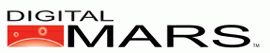D Programming Language 1.0

Last update Mon Dec 31 10:53:28 2012

# std.bitarray

Source:
std/bitarray.d

struct BitArray;
An array of bits.

bool opIndex(size_t i);
bool opIndexAssign(bool b, size_t i);
Support for [index] operation for BitArray.

BitArray dup();
Support for array.dup property for BitArray.

int opApply(int delegate(ref bool) dg);
int opApply(int delegate(ref uint, ref bool) dg);
Support for foreach loops for BitArray.

BitArray reverse();
Support for array.reverse property for BitArray.

BitArray sort();
Support for array.sort property for BitArray.

int opEquals(BitArray a2);
Support for operators == and != for bit arrays.

int opCmp(BitArray a2);
Implement comparison operators.

void init(bool[] ba);
Set BitArray to contents of ba[]

void init(void[] v, size_t numbits);
Map BitArray onto v[], with numbits being the number of bits in the array. Does not copy the data.

This is the inverse of opCast.

void[] opCast();
Convert to void[].

BitArray opCom();
Support for unary operator ~ for bit arrays.

BitArray opAnd(BitArray e2);
Support for binary operator & for bit arrays.

BitArray opOr(BitArray e2);
Support for binary operator | for bit arrays.

BitArray opXor(BitArray e2);
Support for binary operator ^ for bit arrays.

BitArray opSub(BitArray e2);
Support for binary operator - for bit arrays.

a - b for BitArrays means the same thing as a & ~b.

BitArray opAndAssign(BitArray e2);
Support for operator &= bit arrays.

BitArray opOrAssign(BitArray e2);
Support for operator |= for bit arrays.

BitArray opXorAssign(BitArray e2);
Support for operator ^= for bit arrays.

BitArray opSubAssign(BitArray e2);
Support for operator -= for bit arrays.

a -= b for BitArrays means the same thing as a &= ~b.

BitArray opCatAssign(bool b);
BitArray opCatAssign(BitArray b);
Support for operator ~= for bit arrays.

BitArray opCat(bool b);
BitArray opCat_r(bool b);
BitArray opCat(BitArray b);
Support for binary operator ~ for bit arrays.